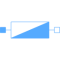# Information

This information is part of the Modelica Standard Library maintained by the Modelica Association.

The admittance model represents a parallel connection of a conductor and either a capacitor or inductor.The linear admittance connects the voltage `v` with the current `i` by `i = Y*v`. The resistive component is modeled temperature dependent, so the real part `G_actual = real(Y)` is determined from the actual operating temperature and the reference input conductance `real(Y_ref)`. A conditional heat port is considered. The reactive component `B_actual = imag(Y)` is equal to `imag(Y_ref)` if `frequencyDependent = false`. Frequency dependency is considered by `frequencyDependent = true`, distinguishing two cases:

(a) `imag(Y_ref) > 0`: capacitive case
The actual susceptance `B_actual` is proportional to `f/f_ref`
(b) `imag(Y_ref) < 0`: inductive case
The actual susceptance `B_actual` is proportional to `f_ref/f`

# Parameters (9)

useHeatPort Value: false Type: Boolean Description: =true, if heatPort is enabled Value: T_ref Type: Temperature (K) Description: Fixed device temperature if useHeatPort = false Value: Type: ComplexAdmittance Description: Complex admittance G_ref + j*B_ref Value: 293.15 Type: Temperature (K) Description: Reference temperature Value: 0 Type: LinearTemperatureCoefficient (¹/K) Description: Temperature coefficient of resistance (R_actual = R_ref*(1 + alpha_ref*(heatPort.T - T_ref)) Value: false Type: Boolean Description: Consider frequency dependency, if true Value: 1 Type: Frequency (Hz) Description: Reference frequency, if frequency dependency is considered Value: real(Y_ref) Type: Conductance (S) Description: Resistive component of conductance Value: imag(Y_ref) Type: Susceptance (S) Description: Reactive component of susceptance

# Connectors (3)

pin_p pin_n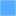Type: PositivePin Description: Positive quasi-static single-phase pin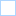Type: NegativePin Description: Negative quasi-static single-phase pin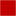Type: HeatPort_a Description: Conditional heat port

# Components (3)

v i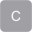Type: ComplexVoltage Description: Complex voltageType: ComplexCurrent Description: Complex currentType: ComplexAdmittance Description: Complex admittance G_ref + j*B_ref

# Used in Components (1)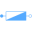Admittance Modelica.Electrical.QuasiStationary.MultiPhase.Basic Multiphase linear admittance